In :
aheights = [6*12+1]*5 + [6*12]*30 + [5*12+11]*5
bheights = [5*12]*20 + [7*12]*20

In :
print 'means:',mean(aheights),mean(bheights)
print 'variances:',var(aheights),var(bheights)
print 'std deviations:',std(aheights),std(bheights)

means: 72.0 72.0
variances: 0.25 144.0
std deviations: 0.5 12.0

In :
figure(figsize=(7,5))
hist(aheights,bins=arange(59.5,90))
hist(bheights,bins=arange(59.5,90))
xlabel('inches'),ylabel('#people with that height')
legend(['mean {}, std {}'.format(mean(d),std(d)) for d in (aheights,bheights)]);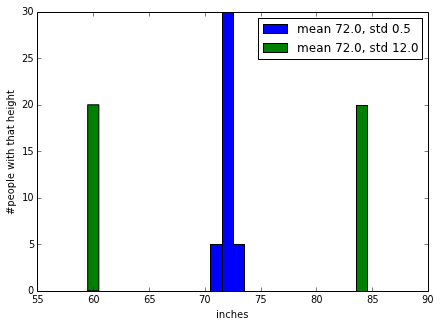In :
from scipy.misc import comb
def probs(N,p=.5):
return array([comb(N,k)*p**k*(1-p)**(N-k) for k in range(N+1)])


In class we discussed calculating the variance of $X$, where $X$ was the value of rolling a single die.
Recall $E[X] = (1+2+3+4+5+6)/6 = 7/2$,
and $E[X^2]= (1^2+2^2+3^2+4^2+5^2+6^2)/6=91/6$,
so $V[X]=E[X^2]-(E[X])^2 = 91/6-49/4=35/12=2.91\overline6$ .

We can compare that to picking random numbers from 1 through 6 using randint(1,7). 10 of these can be generated either via [randint(1,7) for t in xrange(10)], or directly via randint(1,7,size=10), e.g.,

In :
randint(1,7,10)

Out:
array([1, 2, 5, 4, 5, 3, 4, 6, 3, 5])

For large numbers of "rolls", each of the six numbers will appear roughly equal numbers of times. The var() function calculates the mean of the data that it's given, then sums the squared differences of the data points from the mean, and divides by the number of data points. For one million rolls of the die, the mean and variance of the distribution are close to the above theoretical values:

In :
data=randint(1,7,1000000)
mean(data),var(data)

Out:
(3.500246, 2.916728)
In :
hcount=[0 for i in range(11)]
trials = 1000
for j in xrange(trials):
flips= randint(0,2,size=10)  #a sequence of 10 coin flips

In :
bar(range(11),hcount,align='center')
plot(trials*probs(10),'ro--')
xticks(range(11))
xlim(-.5,10.5)
ylabel('#trials with that #heads ('+str(trials)+' total)')
legend(['predicted','counts'])
#;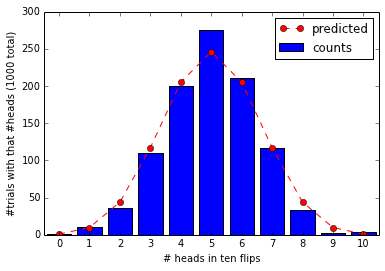In :
zip([round(x,3) for x in trials*probs(10)],hcount)

Out:
[(0.977, 1),
(9.766, 11),
(43.945, 36),
(117.188, 110),
(205.078, 200),
(246.094, 275),
(205.078, 211),
(117.188, 117),
(43.945, 33),
(9.766, 2),
(0.977, 4)]
In :
randint(0,2,size=10)

Out:
array([0, 0, 1, 1, 0, 1, 1, 0, 0, 1])
In :
trials,flips=100,10
title('{} trials of {} flips'.format(trials,flips))
xlim(-.5,10.5),xticks(range(11))
#        same thing in one line of code
hist([sum(randint(0,2,size=10)) for j in xrange(trials)], bins=arange(-.5,11));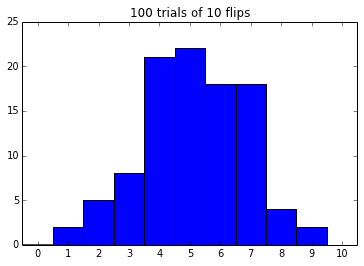In :
#now switch to trials of 100 flips
trials = 1000
results=[sum(randint(0,2,size=100)) for j in xrange(trials)]

In :
results[:10]   #first ten results

Out:
[53, 43, 52, 51, 53, 50, 50, 48, 53, 55]
In :
hist(results,bins=range(101))
ylabel('#trials with that #heads ('+str(trials)+' total)')
#;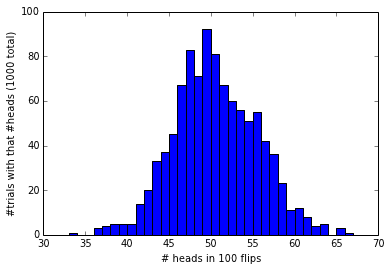In :
std(results),sqrt(100./4)  #compare Var to N*p*(1-p)

Out:
(5.0221365971068623, 5.0)
In :
mean(results)

Out:
50.012
In :
x=randint(1,7,size=10) #now roll a die ten times

In :
print x,', sixes:', sum(x==6)

[5 6 2 5 2 1 4 2 6 5] , sixes: 2

In :
x==6    # tests whether members of array are equal to 6, True=1

Out:
array([False,  True, False, False, False, False, False, False,  True, False], dtype=bool)
In :
def sixes(rolls=100):
rolls=randint(1,7,size=rolls) #default to 100 rolls
return sum(rolls==6)  # return the number of sixes

trials = 1000
results = [sixes(100) for j in xrange(trials)]

In :
hist(results,bins=range(101))
xlabel('# sixes in 100 rolls of die')
ylabel('#trials with that #sixes ('+str(trials)+' total)')
#;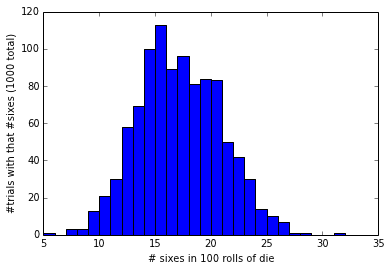In :
print 'mean',mean(results),100./6
print 'std',std(results),sqrt(100*(1/6.)*(5/6.)) #compare to N*p(1-p)

mean 16.683 16.6666666667
std 3.73182408481 3.7267799625


Postscript 1: A local python guru reminded me that randint can produce a two dimensional array, so another way of accumulating statistics for the number of heads in 1000 trials of 100 flips is (where .sum(1) sums along the rows):

In :
trials,flips=1000,100
hist(randint(0,2,(trials,flips)).sum(1),arange(flips+1));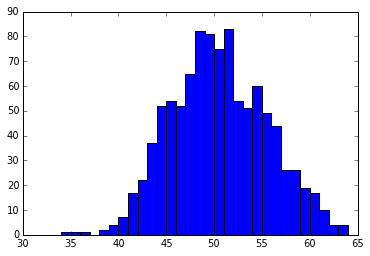Now consider tossing a coin 100 times, see what is the max length string of Heads or Tails

In :
from random import random
import re
from collections import Counter

In :
tosses= ''
for i in range(100):
if random() >= .5:
tosses += 'H'
else:
tosses += 'T'

In :
tosses

Out:
'THHHTHTTTTTTTHTTHTTTHHHHTHHTTTTHHHTTTHHTHTTTTTHTHTHHTTHHTHHTTHHHTHTHHTHTTTHTHTTHHHTHHHHHTHTTTTTTTTHH'
In :
re.findall('H+|T+',tosses)[:12]

Out:
['T', 'HHH', 'T', 'H', 'TTTTTTT', 'H', 'TT', 'H', 'TTT', 'HHHH', 'T', 'HH']
In :
map(len,re.findall('H+|T+',tosses))[:12]

Out:
[1, 3, 1, 1, 7, 1, 2, 1, 3, 4, 1, 2]
In :
max(map(len,re.findall('H+|T+',tosses)))

Out:
8
In :
def maxlength():
tosses= ''
for i in xrange(100):
if random() >= .5:
tosses += 'H'
else:
tosses += 'T'
return max(map(len,re.findall('H+|T+',tosses)))

In :
trials = 10000
results = [maxlength() for t in xrange(trials)]

In :
results[:10]

Out:
[8, 7, 8, 5, 6, 7, 6, 7, 5, 6]
In :
max(results)

Out:
22
In :
counts=Counter(results)

In :
counts

Out:
Counter({6: 2700, 7: 2279, 5: 1667, 8: 1442, 9: 808, 10: 427, 4: 259, 11: 220, 12: 100, 13: 48, 14: 16, 15: 14, 16: 11, 3: 4, 17: 2, 18: 2, 22: 1})
In :
[(x,counts[x]) for x in sorted(counts)]

Out:
[(3, 4),
(4, 259),
(5, 1667),
(6, 2700),
(7, 2279),
(8, 1442),
(9, 808),
(10, 427),
(11, 220),
(12, 100),
(13, 48),
(14, 16),
(15, 14),
(16, 11),
(17, 2),
(18, 2),
(22, 1)]
In :
figure(figsize=(8,6))
hist(results,bins=range(25))
xticks(arange(.5,25.5),range(25));
xlabel('max length string of heads or tails')
ylabel('occurred # times in '+str(trials)+' trials')
#;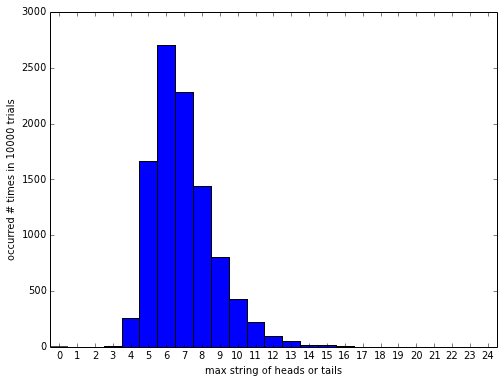In :
cumul=cumsum([counts[i] for i in range(max(counts),-1,-1)])[::-1]

In :
print trials,'trials of 100 flips'
print 'length k',' '*10,'prob longest:'
print ' '*10,'at least k',' '*10, 'exactly k'
for i in range(min(counts),max(counts)+1):
if i not in counts: counts[i] = 0
print '{:>2}            {:<20} {:<6}'.format(i,cumul[i]/float(trials),
counts[i]/10000.)

10000 trials of 100 flips
length k            prob longest:
at least k            exactly k
3            1.0                  0.0004
4            0.9996               0.0259
5            0.9737               0.1667
6            0.807                0.27
7            0.537                0.2279
8            0.3091               0.1442
9            0.1649               0.0808
10            0.0841               0.0427
11            0.0414               0.022
12            0.0194               0.01
13            0.0094               0.0048
14            0.0046               0.0016
15            0.003                0.0014
16            0.0016               0.0011
17            0.0005               0.0002
18            0.0003               0.0002
19            0.0001               0.0
20            0.0001               0.0
21            0.0001               0.0
22            0.0001               0.0001


Postscript 2: The same local python guru pointed out a one-liner for the above statistics as well (this may be a bit obscure, but the abs(diff ( )) is 1 in the places where there's a transition from 0 to 1 or 1 to 0, the where finds the location of those places in the array, and the outer diff then finds the distances between the transition points, i.e., the length of the run of 1's or 0's):

In :
hist([ max(diff( where(abs(diff(randint(0,2,100)))==1) )) for j in xrange(10000)],arange(-.5,30));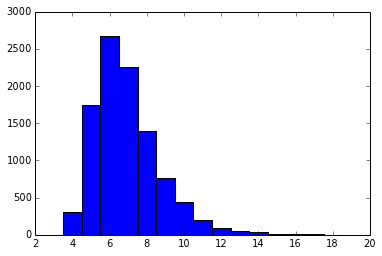In [ ]: